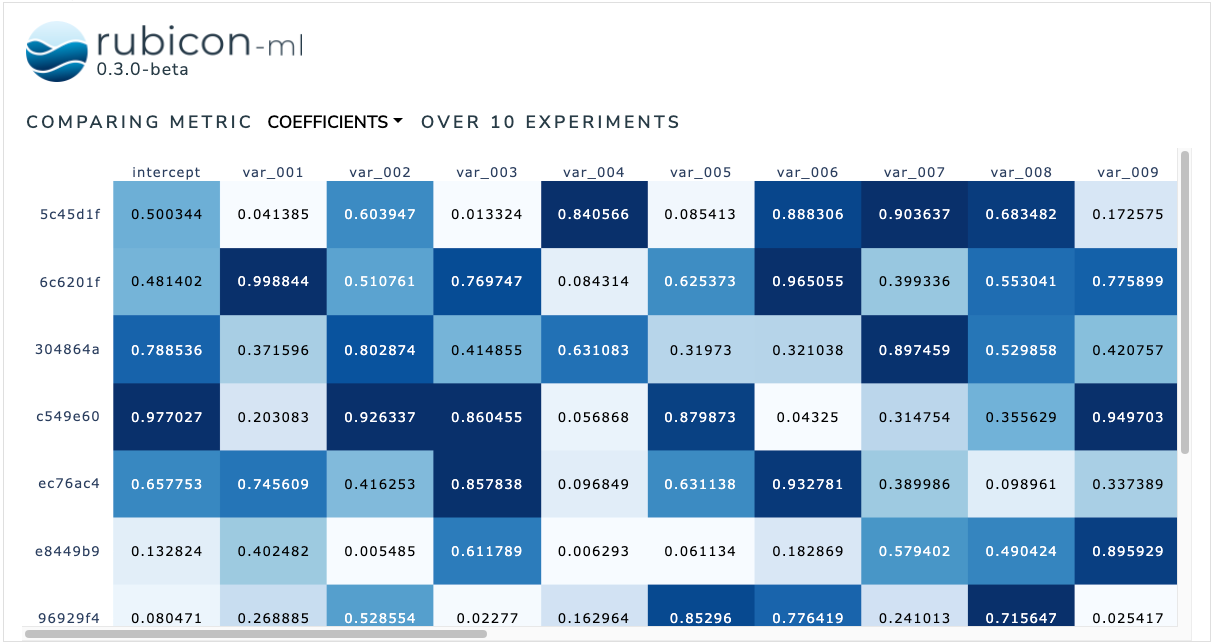# Metric Lists Comparison#

The metric lists comparison is used to facilitate element-wise comparisons between metrics logged as lists. Users can dynamically choose between available list metrics to visualize.

The plot itself is a Plotly annotated heatmap. More information can be found in the Plotly documentation.

:

import random

from rubicon_ml import Rubicon
from rubicon_ml.viz import MetricListsComparison


First, we’ll create a few experiments and log some list metrics to them.

:

rubicon = Rubicon(persistence="memory", auto_git_enabled=True)
project = rubicon.get_or_create_project("list metric comparison")

for i in range(0, 10):
experiment = project.log_experiment()
experiment.log_metric(
name="coefficients",
value=[random.random() for _ in range(0, 25)],
)
experiment.log_metric(
name="p-values",
value=[random.random() for _ in range(0, 25)],
)
experiment.log_metric(
name="stderr",
value=[random.random() for _ in range(0, 25)],
)


Now, we can instantiate the MetricListsComparison object with the experiments we just logged. Optionally, provide a list of column names as column_names to add the names as a header to each column in the visualization.

We can view the plot right in the notebook with show. The Dash application itself will be running on http://127.0.0.1:8050/ when running locally. Use the serve command to launch the server directly without rendering the widget in the current Python interpreter.

:

MetricListsComparison(
experiments=project.experiments(),
column_names=["intercept"] + [f"var_{i:03}" for i in range(1, 25)],
).show()

Dash is running on http://127.0.0.1:8050/